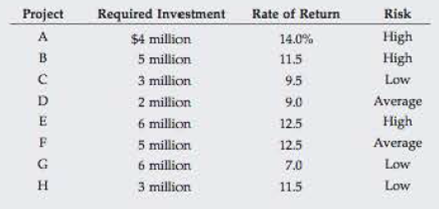Chapter 10, Problem 19P### Fundamentals of Financial Manageme...

9th Edition
Eugene F. Brigham + 1 other
ISBN: 9781305635937

#### Solutions

Chapter
Section### Fundamentals of Financial Manageme...

9th Edition
Eugene F. Brigham + 1 other
ISBN: 9781305635937
Textbook Problem
10 views

# ADJUSTING COST OF CAPITAL FOR RISK Ziege Systems is considering the following independent projects for the coming year:Ziege’s WACC is 10%, but it adjusts for risk by adding 2% to the WACC for high-risk projects and subtracting 2% for low-risk projects. a. Which projects should Ziege accept if it faces no capital constraints? b. If Ziege can only invest a total of $13 million, which projects should it accept, and what would be the dollar size of its capital budget? c. Suppose Ziege can raise additional funds beyond the$13 million, but each new increment (or partial increment) of \$5 million of new capital will cause the WACC to increase by 1%. Assuming that Ziege uses the same method of risk adjustment, which projects should it now accept, and what would be the dollar size of its capital budget?

a.

Summary Introduction

To identify: The project Z Systems should accept if its faces no capital constraints.

Introduction:

The firms should consider the risk associated with a particular project. The risk is high or low in the project should be checked and the WACC should be adjusted. Thus risk adjusted WACC is used to assess projects.

Explanation

The following projects should be accepted.

 Project Required Investment (in million dollars) Rate of Return (%) Risk Adjusted WACC H 3 11.5 Low 10%−2%=8% C 3 9.5 Low 10%−2%=8% F 5 12.5 Average 10% A 4 14.0 High 10%+2%=12% E 6 12.5 High 10%+2%=12%

Table (1)

Working note:

Computation of adjusted WACC of individual projects

 Project Required Investment (in million dollars) Rate of Return (%) Risk Adjusted WACC A 4 14

b.

Summary Introduction

To identify: The projects that should be accepted by Z system and the dollar size of its capital project.

c.

Summary Introduction

To determine: The project should be accepted by the company now and the dollar size of its capital budget.

### Still sussing out bartleby?

Check out a sample textbook solution.

See a sample solution

#### The Solution to Your Study Problems

Bartleby provides explanations to thousands of textbook problems written by our experts, many with advanced degrees!

Get Started